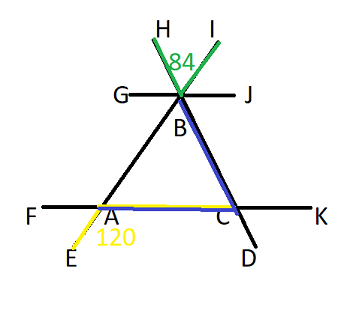# If angle EAC is 120 degrees and angle HBI is 84 degrees, what is the angle of BCA? I need help...

## Question:

If angle EAC is 120 degrees and angle HBI is 84 degrees, what is the angle of BCA?

## Triangles:

There are different types of triangle. Isosceles, obtuse and scalene are three types of triangles, yet if you add the inner angels of any triangle the total always comes to 180.

Become a Study.com member to unlock this answer!

If angle EAC is 120 degrees and angle HBI is 84 degrees, the angle of BCA is 36 degrees.Given that straight lines...Types of Angles: Vertical, Corresponding, Alternate Interior & Others

from

Chapter 14 / Lesson 9
268K

What are the types of angles and angle relationships? Learn about corresponding interior angles, alternate corresponding angles, and angle relationships names.